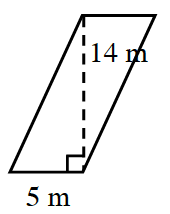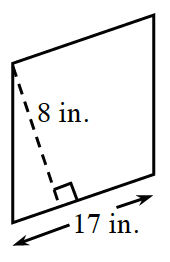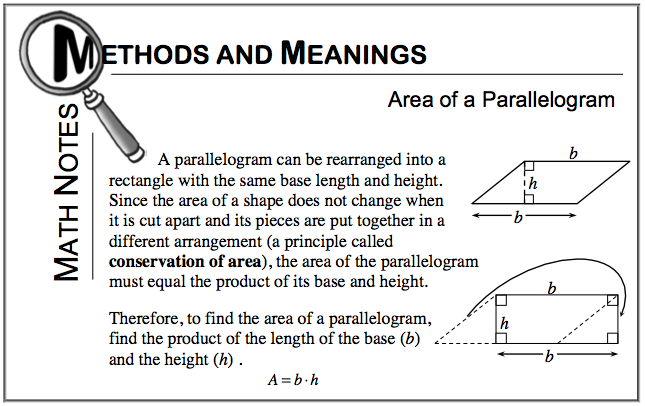### Home > CC1 > Chapter 5 > Lesson 5.3.3 > Problem5-91

5-91.

Find the area of each parallelogram below. Show all of your work. Use the Math Notes box in this lesson if you need help. Homework Help ✎

See the Math Notes box at the bottom of the page.

1.As the Math Notes box says, the area of the parallelogram is equal to the product of its base and its height. Thus, multiply 5(14) to find this parallelogram’s area.

(5 m)(14 m) = 70 m2

1.If you rotate the figure in part (b), you will see that the base is 17 in. and the height is 8 in.